# CLASS 12 PHYSICS CHAPTER 10-WAVE OPTICS

Question1. Monochromatic light having a wavelength of 589nm from the air is incident on a water surface. Find the frequency, wavelength and speed of (i) reflected and (ii) refracted light? [1.33 is the Refractive index of water]

Solution :
Monochromatic light incident having wavelength, λ = 589 nm = 589 x 10-9 m

Speed of light in air, c = 3 x 108 m s-1

Refractive index of water, μ = 1.33

(i) In the same medium through which incident ray passed the ray will be reflected back.

Therefore the wavelength, speed, and frequency of the reflected ray will be the same as that of the incident ray.

Frequency of light can be found from the relation:

Hence, the speed, frequency, and wavelength of the reflected light are:

c = 3 x 108 m s-1, 5.09 × 1014 Hz, and 589 nm respectively.

(b) The frequency of light which is travelling never depends upon the property of the medium. Therefore, the frequency of the refracted ray in water will be equal to the frequency of the incident or reflected light in air.

Refracted frequency, v = 5.09 x 1014 Hz

Speed of light in water Is related to the refractive Index of water as:

Wavelength of light in water is given by the relation,

Hence the speed, frequency and wavelength of refracted light are: 444.007 × 10-9 m, 444.01nm, and 5.09 × 1014 Hz respectively.

Question2. What is the shape of the wavefront in each of the following cases:

(a) Light diverging from a point source.

(b) Light emerging out of a convex lens when a point source is placed at its focus.

(c) The portion of the wavefront of light from a distant star intercepted by the Earth.

Solution :
(a) The shape of the wavefront in case of a light diverging from a point source is spherical. The wavefront emanating from a point source is shown in the given figure.

(b) The shape of the wavefront in case of a light emerging out of a convex lens when a point source is placed at its focus is a parallel grid. This is shown in the given figure.

(c) The portion of the wavefront of light from a distant star intercepted by the Earth is a plane.

Question3. (a) The refractive index of glass is 1.5. What is the speed of light in glass? Speed of light in vacuum is 3.0 × 108 m s−1)

(b) Is the speed of light in glass independent of the colour of light? If not, which of the two colours red and violet travels slower in a glass prism?

Solution :
(a) Refractive index of glass, μ = 1.5

Speed of light, c = 3 × 108 m/s

Speed of light in glass is given by the relation,

Hence, the speed of light in glass is 2 × 108 m/s.

(b) The speed of light in glass is not independent of the colour of light. The refractive Index of a violet component of white light is greater than the refractive Index of a red component. Hence, the speed of violet light is less than the speed of red light in glass. Hence, violet light travels slower than red light in a glass prism.

Question4. In a Young’s double-slit experiment, the slits are separated by 0.28 mm and the screen is placed 1.4 m away. The distance between the central bright fringe and the fourth bright fringe is measured to be 1.2 cm. Determine the wavelength of light used in the experiment.

Solution :
Distance between the slits, d = 0.28 mm = 0.28 × 10−3 m

Distance between the slits and the screen, D = 1.4 m

Distance between the central fringe and the fourth (n = 4) fringe,

u = 1.2 cm = 1.2 × 10−2 m

In case of a constructive interference, we have the relation for the distance between the two fringes as:

Where,

n = Order of fringes = 4

λ = Wavelength of light used

Hence, the wavelength of the light is 600 nm.

Question5. In Young’s double-slit experiment using monochromatic light of wavelengthλ, the intensity of light at a point on the screen where path difference is λ, is K units. What is the intensity of light at a point where path difference is λ /3?

Solution :
Let I1 and I2 be the intensity of the two light waves. Their resultant intensities can be obtained as:

Where,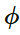= Phase difference between the two waves

For monochromatic light waves,

Phase difference =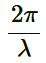Since path difference = λ,

Phase difference,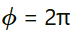Given,

I’ = K

When path difference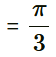Phase difference,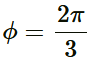Hence, resultant intensity,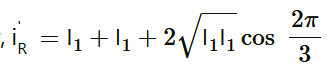Using equation (1), we can write:

Hence, the intensity of light at a point where the path difference is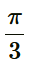is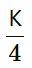units.

Question6. A beam of light consisting of two wavelengths, 650 nm and 520 nm, is used to obtain interference fringes in a Young’s double-slit experiment.

(a) Find the distance of the third bright fringe on the screen from the central maximum for wavelength 650 nm.

(b) What is the least distance from the central maximum where the bright fringes due to both the wavelengths coincide?

Solution :
Wavelength of the light beam,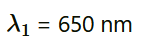Wavelength of another light beam,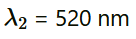Distance of the slits from the screen = D

Distance between the two slits = d

(a) Distance of the nth bright fringe on the screen from the central maximum is given by the relation,

(b) Let the nth bright fringe due to wavelength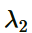and (n − 1)th bright fringe due to wavelength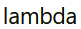coincide on the screen. We can equate the conditions for bright fringes as:

Hence, the least distance from the central maximum can be obtained by the relation:

Question7. In a double-slit experiment the angular width of a fringe is found to be 0.2° on a screen placed 1 m away. The wavelength of light used is 600 nm. What will be the angular width of the fringe if the entire experimental apparatus is immersed in water? Take refractive index of water to be 4/3.

Solution :
Distance of the screen from the slits, D = 1 m

Wavelength of light used,Angular width of the fringe in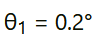Angular width of the fringe in water =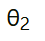Refractive index of water,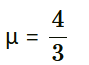Refractive index is related to angular width as:

Therefore, the angular width of the fringe in water will reduce to 0.15°.

Question8. What is the Brewster angle for air to glass transition? (Refractive index of glass = 1.5.)

Solution :
Refractive index of glass,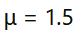Brewster angle = θ

Brewster angle is related to refractive index as:

Therefore, the Brewster angle for air to glass transition is 56.31°.

Question9. Light of wavelength 5000 Å falls on a plane reflecting surface. What are the wavelength and frequency of the reflected light? For what angle of incidence is the reflected ray normal to the incident ray?

Solution :
Wavelength of incident light, [λ] = 5000 Armstrong = 5000 x 10-10 m

Speed of light, c =3 x 108 m

Frequency of incident light is given by the relation,

The wavelength and frequency of incident light is the same as that of reflected ray. Hence, the wavelength of reflected light is 5000 Armstrong and its frequency is 6×1014Hz. When reflected ray is normal to incident ray, the sum of the angle of incidence, ∠i and angle of reflection, ∠r is 90°

According to the law of reflection, the angle of incidence is always equal to the angle of reflection. Hence, we can write the sum as:

∠i+∠r = 90°

i.e. ∠i+∠i = 90°

Hence, ∠i=902 = 45°

Therefore, the angle of incidence for the given condition is 45°

Question10. Estimate the distance for which ray optics is good approximation for an aperture of 4 mm and wavelength 400 nm.

Solution :
Fresnel’s distance (ZF) is the distance for which the ray optics is a good approximation. It is given by the relation,

ZF=a2λ

Where,

Aperture width, a = 4 mm = 4 × 10-3 m

Wavelength of light, λ = 400 nm = 400 × 10-9 m

Therefore, the distance for which the ray optics is a good approximation is 40 m.

Get 30% off your first purchase!

X
error: Content is protected !!
Scroll to Top